#### How to Calculate Difference in Excel

In this Excel lesson you will teach yourself a lot about difference any kind in Excel.

## Difference between two dates

The easiest way to calculate date difference is to use DATEDIF function. This is dedicated function to calculate difference between two dates.

=DATEDIF(B1,B2,”D”)formula to calculate days between dates

=DATEDIF(B1,B2,”M”) – formula to calculate months between dates

=DATEDIF(B1,B2,”Y”) – formula to calculate years between dates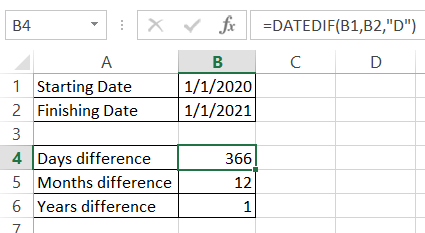There is also a possibility to calculate quarters between dates.

## Difference between two columns

You have two columns and would like to compare them. This is possible with Excel.

First thing you can check is if the cells are exactly the same. It is a Excel formula which easily spot the difference.

=IF(\$A2=\$B2,”MATCH”,”DIFFERENCE SPOTTED”)

We used IF function to write the result down.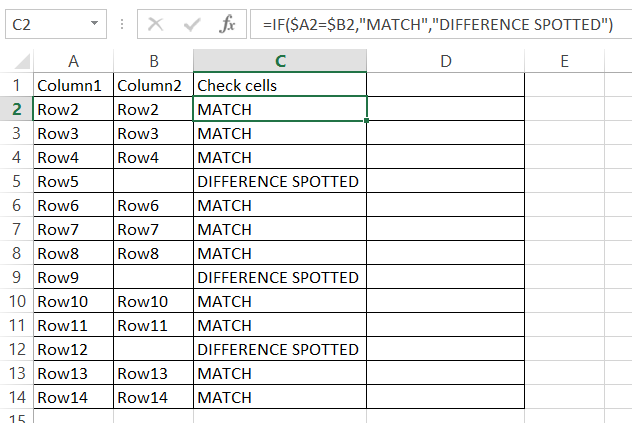The second way is to check if the cell value exists in the other column. Vlookup function knowledge will help to build such Excel formula: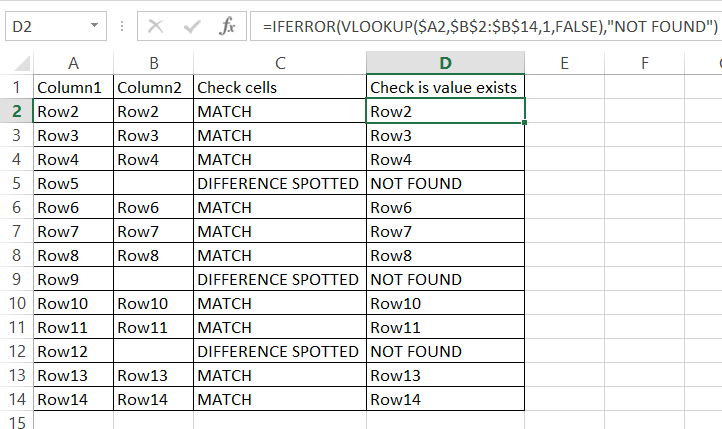You can also compare columns for duplicates.

## How do I compare time differences in Excel?

Good question! There is no dedicated Excel function for time difference. Instead of that just use simple subtraction and properly configure your data formatting.

See the picture. I used simple Excel subtraction.

=TEXT(\$B2-\$A2,”HH:MM:SS”)

The trick is to change data formatting. Instead of doing it meanually I used TEXT function to do that. I will get the result as hours, minutes and seconds.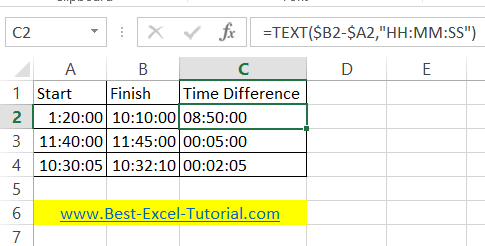Please check laso time math tutorial.

## Percentage difference

To calculate the percentage difference you need to remember to subtract 1. Excel formula will be:

=\$B2/\$A2-1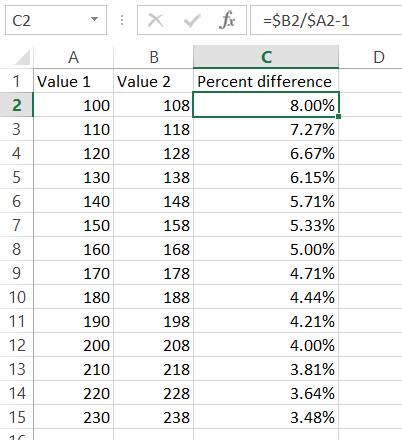Subtracting 1 is needed to see the percentage difference.

More on how to calculate percent change in excel here.

Note that you can also use the ABS function to ensure that the result is always positive. For example, if you want to find the difference between the values in cell A1 and B1, and you want the result to always be positive, you can use the formula “=ABS(B1-A1)”.

Here you can find spreadsheet used in the lesson.